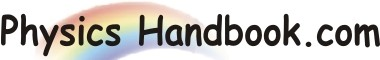HOME TOPICS DEFINITIONS TABLES LAWS INVENTIONS EXPERIMENTS QUIZ VIDEOS
 Dalton's Law Dalton's Law states that the sum of the partial pressures of the individual gases of a gas mixture is equal to the total pressure of the mixture. i.e   PressureTotal  =  P1 + P2... + Pn Where, P1, P2, Pn represents the partial pressures of the individual gases. In general, P  =  ΣPi Where, P = Total pressure of the mixture Pi = Partial pressures of the individual gases